# Ranked choice voting is a system of tallying election ballots that is used in many national...

Ranked choice voting is a system of tallying election ballots that is used in many national and local elections throughout the world. Instead of choosing a single candidate, voters must rank the available candidates in the order of their choice. For example, if three candidates are available, a voter might choose #2, #1, and #3 as their choices, with #2 being their first choice, #1 the second, and #3 the third.

The outcome is determined by a runoff, which follows this process:

1) The first choices on all ballots are counted. If one candidate receives more than 50% of the vote, that candidate is elected.

2) If no candidate receives more than 50% of the vote, the lowest scoring candidate is eliminated (or multiple candidates if there is a tie for lowest scoring).

3) The ballots are recounted, using the highest ranking non-eliminated candidate on each ballot.

4) The process is repeated until one candidate receives more than 50% of the vote or there is a tie.

Here is a good video describing the process visually:

Ranked Choice Voting!?! Here's How it Works (Links to an external site.)Assignment

Write a program that performs a series of ranked choice elections based on an input file.

Each election contains 3 candidates. The input file will contain multiple elections, in the following format:

1) At the beginning of a test case, a single integer indicating the number of lines that follow.

2) A series of 3-line ballots, with the voter's first choice being on the first line, second choice on the second, and third on the last line. In this

3) There can be up to 500 ballots in an election and no less than 5.

For example, the following file has 3 ballots:

```9
3
1
2
1
2
3
3
2
1```

The first ballot selects candidates 3, 1 and 2 in that order. The second ballot selects candidates 1, 2 and 3 in that order. Similarly the third selects candidates 3, 2, and 1.

Requirements

1) For each test case, output the number of ballots read and the winner (something like "Candidate #1 wins").

2) You are free to use any parts of the C++ language we've studied in this course.

3) Dynamic memory is not necessary, but you may find it useful to to allocate the buffer used to hold the ballots dynamically. For example, if you anticipate 300 ballots, allocate an array of 900 lines (3 lines for each ballot).

4) The program must read the input file and tally all votes according to a ranked choice system. No hard-coded inputs or outputs will be accepted.

5) Read the file continuously until EOF and process all elections in the file. After EOF, the program can exit. It is OK to hard code the filename (i.e. not ask the user for input).

Input File

A file containing 3 test cases is posted here -- elections.txt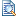-- use this as the input to test your program. Edit: I cant find the download to the txt file but here are the numbers that are in elections.txt:

15

1

2

3

3

2

1

2

1

3

1

2

3

2

3

1

15

1

2

3

1

2

3

1

2

3

1

2

3

2

1

3

15

3

2

1

3

2

1

3

1

2

1

2

3

1

3

2

Example Output

```Read 5 ballots.
Candidate #2 wins.

Candidate #1 wins.

Candidate #3 wins.```
We were unable to transcribe this image
We were unable to transcribe this image

BELOW IS THE IMPLEMENTATION OF ABOVE PROBLEM ,FILE NAME IS ELECTIONS.TXT , PLEASE READ THE FILE WITH SAME NAME FROM THE SAME DIRECTORY OF THE PROJECT.I MADE IT USING CODE BLOCKS COMPILER.

IT TAKES INPUT FILE AND READ LINE BY LINE INTEGERS AND CORRESPONDINGLY CALCULATES THE RESULT,PLEASE GO THROUGH THE CODE ONCE (OR MORE) TO GET A CLEAR IDEA OF WHAT I HAVE DONE.

AND PLEASE SUGGEST ANY IMPROVEMENT AND IF YOU DO NOT UNDERSTAND ANYTHING IN THE CODE PLEASE COMMENT , I WILL SOLVE IT FOR YOU.

AND IF YOU LIKE IT PLEASE UPVOTE,THANK YOU.

FOR THE CUSTOM INPUT PROVIDED THE OUTPUT LOOKS LIKE THIS: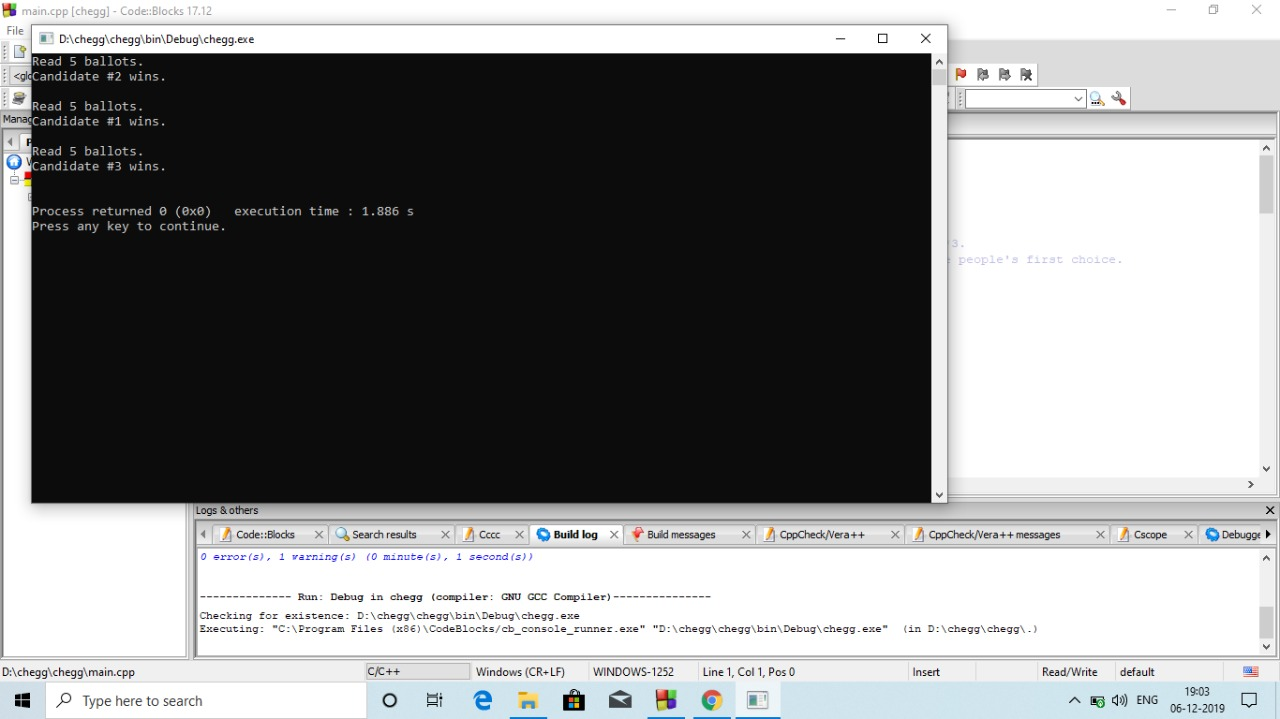#include<bits/stdc++.h>
using namespace std;
int main(){
ifstream theFile("elections.txt");//file pointer
int x;
while(theFile >> x){// reading integer from file line to line.
int total=x;// first input number is total number of vote i.e, number of ballots*3.
map<int,int>arr;// to store frequency of each vote to candidate accordance to the people's first choice.
vector< vector<int> >ve;// vector to store each ballot of size 3
total/=3;// as each ballot is of size 3
int ballots=total;
ve.resize(ballots);
for(int i=0;i<ballots;i++){
ve[i].resize(3);
}
int j=0;
while(total--){// taking all ballot input.
bool f=theFile>>x;

ve[j]=x;

f=theFile>>x;

ve[j]=x;
f=theFile>>x;

ve[j]=x;
j++;
}
bool flag=0;
while(!flag){// flag is set when we elect a candidate
arr.clear();
int sum=0;
for(int i=0;i<ballots;i++){
if(!(ve[i].empty())){
sum+=1;
arr[ve[i]]++;
}
}
int maxi=INT_MIN;
int mini=INT_MAX;
map<int,int>::iterator it;
it=arr.begin();
for(;it!=arr.end();it++){// finding maximum and minimum vote in the people's preferable candidate.
maxi=max(maxi,it->second);
mini=min(mini,it->second);
}
if(maxi>(sum/2.0)){// condition for a candidate to be elected(more than 50%.)
if(maxi==arr){
cout<<"Candidate #"<<1<<" wins."<<"\n";
}
else if(maxi==arr){
cout<<"Candidate #"<<2<<" wins."<<"\n";
}
else{
cout<<"Candidate #"<<3<<" wins."<<"\n";
}
flag=1;
}
else{// if any candidate has minimum number of votes then they are eliminated from further process.
int eliminated;
if(mini==arr){
eliminated=1;
for(int i=0;i<ballots;i++){
vector<int>::iterator vit=ve[i].begin();
for(;vit!=ve[i].end();vit++){
if(*vit==eliminated){
break;
}
}
if(vit!=ve[i].end()){
ve[i].erase(vit);
}
}
}
if(mini==arr){
eliminated=2;
for(int i=0;i<ballots;i++){
vector<int>::iterator vit=ve[i].begin();
for(;vit!=ve[i].end();vit++){
if(*vit==eliminated){
break;
}
}
if(vit!=ve[i].end()){
ve[i].erase(vit);
}
}
}
if(mini==arr){
eliminated=3;
for(int i=0;i<ballots;i++){
vector<int>::iterator vit=ve[i].begin();
for(;vit!=ve[i].end();vit++){
if(*vit==eliminated){
break;
}
}
if(vit!=ve[i].end()){
ve[i].erase(vit);
}
}
}
}
}
cout<<endl;
}
}
// code contributed by MIHIR SINGH codechef:(kassutta)

#### Earn Coin

Coins can be redeemed for fabulous gifts.

Similar Homework Help Questions
• ### C++ ☆ HackLand Election In elections that use the ballot box system for voting, each voter...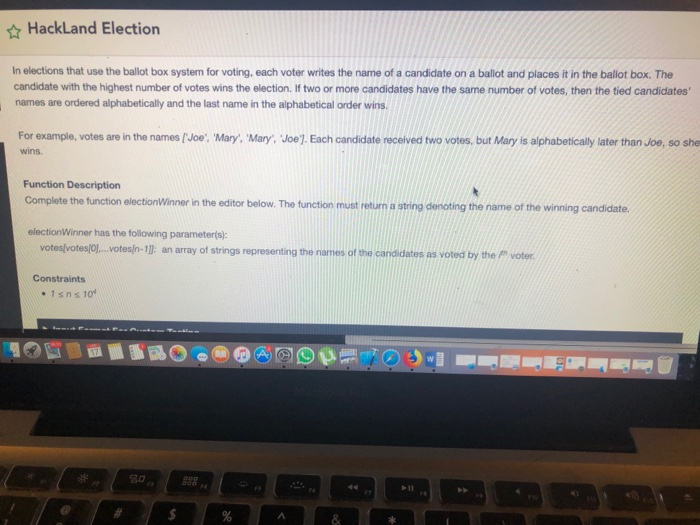C++ ☆ HackLand Election In elections that use the ballot box system for voting, each voter writes the name of a candidate on a ballot and places it in the ballot box. The candidate with the highest number of votes wins the election. If two or more candidates have the same number of votes, then the tied candidates' names are ordered alphabetically and the last name in the alphabetical order wins. For example, votes are in the names [Joe, Mary,...

• ### To combat election fraud, your city is instituting a new voting procedure. The ballot has a...

To combat election fraud, your city is instituting a new voting procedure. The ballot has a letter associated with every selection a voter may make. A sample ballot is shown:- (Voter places a tick next to his or her preferred candidate, proposition and measure to indicate his/her vote) 1. Mayoral Candidates     A. Pincher, Penny     B. Dover, Skip     C. Perman, Sue 2. PROP 17     D. YES     E. NO 3. MEASURE 1     F. YES     G....

• ### Using C language, write a program for the following: In a student representative council election, there...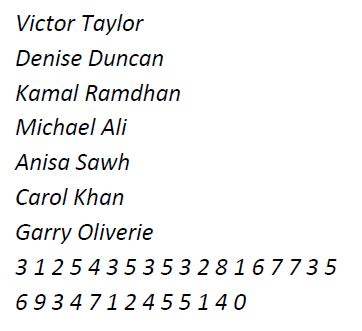Using C language, write a program for the following: In a student representative council election, there are ten (10) candidates. A voter is required to vote a candidate of his/her choice only once. The vote is recorded as a number from 1 to 10. The number of voters are unknown beforehand, so the votes are terminated by a value of zero (0). Votes not within and not inclusive of the numbers 1 to 10 are invalid (spoilt) votes. A file,...

• ### Elections between Hillary and Trump. There are only three voters and all of them strictly prefer...

Elections between Hillary and Trump. There are only three voters and all of them strictly prefer Hilary to Trump. We assume that voters' utility only depends on the candidate that wins the election. In the first round, all voters vote simultaneously. If a candidate is voted by all the three voters, this candidate is elected. If none of the two candidates receives all the votes, then a second round takes place. All voters vote again simultaneously, but the winner is...

• ### INPUT FILE INTO ARRAY. CHECKING FOR COMMAS AND SUCH. HOW TO DO? PLEASE READ CAREFULLY. MAX_SIZE...

INPUT FILE INTO ARRAY. CHECKING FOR COMMAS AND SUCH. HOW TO DO? PLEASE READ CAREFULLY. MAX_SIZE is size of array When iterating over the candidates’ list, do not iterate over the entire array, but just over the records where data is filled in void readFile(Candidate candidates[]) – reads the elections.txt file, fills the candidates[] array. Hint: use substr() and find() functions. Set Score to 0. void List(Candidate candidates[]) – prints the array of Candidate structs. One candidate per one line,...

• ### Question 3 alues, and a get method for each of the member variables. The class implements...Question 3 alues, and a get method for each of the member variables. The class implements the ne member variables to the given Comparable interface, and compares two candidates based on their numbers of votes. 2. Designing a class named VotingMachine that contains a collection of candidates and the following methods: . addCandidate (String name): Add a candidate of a given name to the collection of candidates. This method throws an exception if a candidate of the given name already...

• ### dont need help on the first two pictures, only need help underatanding these: number 1,2,3,4,5,6. please help:/...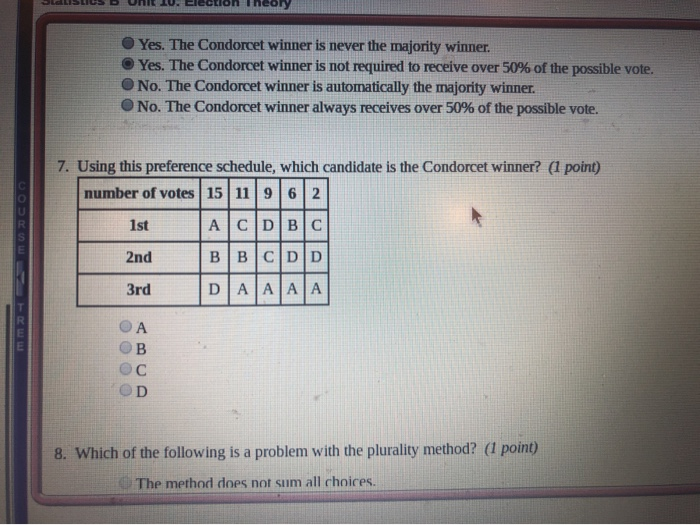dont need help on the first two pictures, only need help underatanding these: number 1,2,3,4,5,6. please help:/ O Yes. The Condorcet winner is never the majority winner Yes. The Condorcet winner is not required to receive over 50% of the possible vote. O No. The Condorcet winner is automatically the majority winner. No. The Condorcet winner always receives over 50% of the possible vote. 7. Using this preference schedule, which candidate is the Condorcet winner? (1 point) number of votes...

• ### Activity Selection Last-to-Start | C++ Implementation Develop a program with a greedy algorithm that instead of always...

Activity Selection Last-to-Start | C++ Implementation Develop a program with a greedy algorithm that instead of always selecting the first activity to finish, instead selects the last activity to start that is compatible with all previously selected activities. The program should read input from a file named “act.txt”. The file contains lists of activity sets with number of activities in the set in the first line followed by lines containing the activity number, start time & finish time. Example act.txt:...

• ### In either Java or Python 3, write a program that simulates a deterministic FSM. It will read from two input files. The first is a file describing an FSM The first line contains the alphabet as a seri...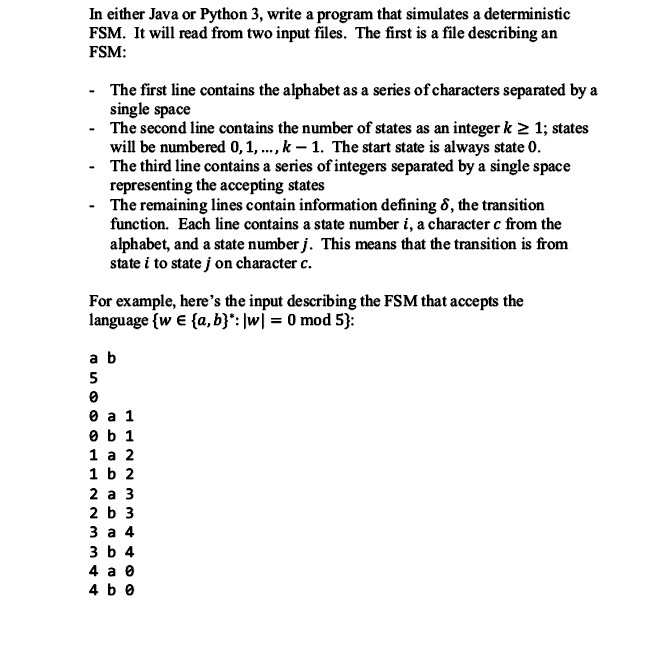In either Java or Python 3, write a program that simulates a deterministic FSM. It will read from two input files. The first is a file describing an FSM The first line contains the alphabet as a series of characters separated by a single space - The second line contains the number of states as an integer k 2 1; states will be numbered 0,1,..., k -1. The start state is always state O The third line contains a series...

• ### In either Java or Python 3, write a program that simulates a deterministic FSM. It will read from two input files. The first is a file describing an FSM The first line contains the alphabet as a seri...In either Java or Python 3, write a program that simulates a deterministic FSM. It will read from two input files. The first is a file describing an FSM The first line contains the alphabet as a series of characters separated by a single space - The second line contains the number of states as an integer k 2 1; states will be numbered 0,1,..., k -1. The start state is always state O The third line contains a series...